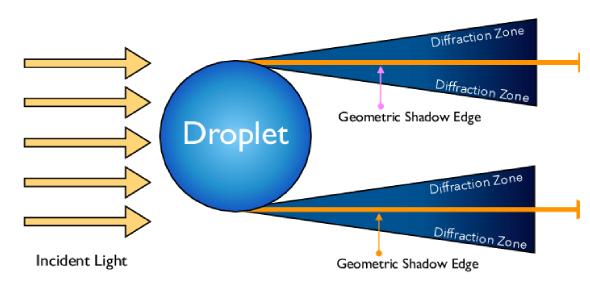# Physical Optics Quiz! Trivia

10 Questions | Total Attempts: 18Settings.

• 1.
A beam of electrons is used in Young's double-slit experiment. If the speed of electrons is increased then the fringe width will:
• A.

Increase

• B.

Decrease

• C.

Remains same

• D.

Fringes will not be seen

• 2.
In YDSE, the slits are 0.05 cm apart and the interference fringes are obtained on a screen 1 m away from the slits. The slits are illuminated by sodium light(5893 A). The distance between 4th bright fringe on one side and 3rd bright fringe on the other side of the central bright fringe is:
• A.

1.25 mm

• B.

5.5 mm

• C.

8.25 mm

• D.

3.78 mm

• 3.
Two slits in YDSE have widths in the ratio 1:25. The ratio of intensity at the maxima and minima in the interference pattern, Imax / Imin:
• A.

121/49

• B.

49/121

• C.

4/9

• D.

9/4

• 4.
The formula for the width of the central maximum in the Fraunhofer diffraction pattern of a single slit as observed on a screen:
• A.

F λ/e

• B.

2λ/e

• C.

F λ/2e

• D.

2f λ/e

• 5.
The angular separation between central maximum and first-order maximum of the diffraction pattern due to a single slit of width 0.25 mm when light of wavelength 5890 A is incident normally on it, is:
• A.

• B.

• C.

• D.

• 6.
In the propagation of light waves, the angle between the direction of propagation and the plane of polarisation is:
• A.

0

• B.

450

• C.

900

• D.

1800

• 7.
A beam of unpolarised light of intensity I0 is passed through a polaroid A and then through another polaroid B which is oriented so that its principal plane makes an angle of 45relative to that of A. The intensity of the emergent light is:
• A.

I0

• B.

I0 /2

• C.

I0 /4

• D.

I0 /8

• 8.
Monochromatic light of wavelength 198 nm is incident on the surface of a metallic cathode, whose work function is 2.5 eV. How much potential difference must be applied between the cathode and the anode of a photocell to just stop the photocurrent from flowing?
• A.

2.55 V

• B.

3.75V

• C.

5.75 V

• D.

1.5 V

• 9.
The work function of a surface of photo sensitive material is 6.2 eV. The wavelength of the incident radiation for which the stopping potential is 5V lies in the?
• A.

Ultraviolet region

• B.

Visible region

• C.

Infrared region

• D.

X- ray region

• 10.
The kinetic energy of a proton is equal to the energy E of a photon. If f1 be the de Broglie wavelength of the proton and f2 that of the photon, then the ratio f1/ f is proportional to:
• A.

E

• B.

E1/2

• C.

--1/2

• D.

--1

Related TopicsBack to top